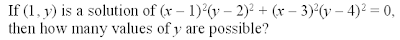## Sunday, July 11, 2010

### Reflections Drawn from SAT Problems

This is the process that I go through whenever I complete one of David Marain's SAT problems of the day:

1. I do the problem. In particular, let me use this one as an example, because it helps to illustrate my point:2. I consider realistically how many of my students would be able to do this problem with reasonable ease/accuracy, and how many kids in general taking the SATs could do this problem. (My uncorroborated rough estimate for this problem is about 45% of all kids, maybe about 30% of my students, and maybe 25% of the kids in general at my school.)

3. I link the problem to a very simple concept that we all already teach in our math classroom -- a concept that is perhaps very pervasive in the high-school curricula. In this case, it is plugging in a solution (or part of a solution) into an equation, because we know that all solutions have to "fit" their equation.

4. I consider the conceptual scaffolding that it would take for the majority of our kids to "get" this problem.

• Figuring out that solution points have to fit the equation.

• Figuring out that you can find the missing coordinate, as long as you are given the equation and the other coordinate. ie. You can find the y-value of (1, y) in this problem by simply plugging in x = 1.

• Having some really basic algebraic skills for simplifying.

• Figuring out that most SAT equations are merely monstrous twists of simple ideas.

• Review this every 6 or so months, for the same concept.

Sounds simple, no? Apparently, something along the line goes awry, because (I believe) more kids than not will likely get this problem wrong on the test. And, why is that the case?? The three first bullet points are basically given. We already do that inside our classroom, don't we?

...Obviously, I do have some less-than-amicable personal feelings about some of these SAT problems. I don't think the way that they are posed supports the juicier things we, as math educators, try to do inside the classroom: helping kids appreciate the meaning behind numbers, creating problems that are inherently interesting, building a connection between intuition and the numeric solution, etc. But, that does not change the fact that I, as a teacher, need to enable my kids to be able to deal with these abstract and intimidating forms in which a simple concept may appear. The SAT problems should not dominate our classroom, but we need to keep in mind that they are in store for our students.*

So, that is one of my goals for next year. I am going to work on my own exposure to SAT problems, and to increasingly sneak those problems in there once the kids have mastered their fear of the concept.

*And I say this for middle-school teachers as well as high-school teachers. I speak from my personal experience only (so maybe this does not reflect other teachers), but we are often so bogged down trying to shoot for fulfilling state standards and covering what seems to be an extensive curriculum, that we skip out on the extra little abstract analysis that could really help our kids down the road on the SATs (and maybe other things)! Is there any reason why this particular problem could not be done with middle-schoolers who have already seen quadratic basics?

1.Once I simplify the equation, using squares, distributive property and combining like terms, I end up with:

2x^2y^2+20x^2+10y^2+148=0

What then?

2.Actually no, i did my squaring wrong , I assumed (x-1)^2 was x^2+1, where it's x^2-2x+1. I still arrive at a rather large polynomial and find myself stuck right there

3.The key of this problem is to NOT expand/simplify and solve (because that gets kind of hairy). The key is to plug in x = 1 as early as possible, to get all the x's to disappear. If you do that right at the beginning of the problem, it immediately becomes:

4(y - 4)^2 = 0

since the first term, (x - 1)^2(y - 2)^2, drops out entirely when you plug in x = 1.

But even if you arrive at a rather large polynomial through expansion, you still need to, at some point, realize that you need to plug in x = 1 in order to find y. Once you do that, you should get a straight-forward quadratic equation equivalent to 4(y - 4)^2 = 0.

Hope that helps! Again, the crux of this problem, for me, is that it is immediately connected to simple ideas, albeit it hairy-looking.